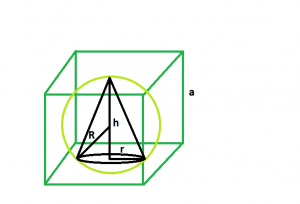# Largest right circular cone that can be inscribed within a sphere which is inscribed within a cube

Given a cube of side length a, which inscribes a sphere which in turn inscribes a right circular cone. The task is to find the largest possible volume of this cone.

Examples:

```Input:  a = 5
Output: 58.1481

Input: a = 8
Output: 238.175
```Approach:
Let, the height of right circular cone = h.
Radius of the cone = r
Radius of the sphere = R
We, know radius of the sphere inside the cube, r = a/2. Please refer ( Largest sphere that can be inscribed inside a cube).
Also, height of cone inside the sphere, h = 4r/3.
radius of cone inside the sphere, r = 2√2r/3. Please refer (Largest right circular cone that can be inscribed within a sphere).
So, height of the cone inside the sphere which in turn is inscribed within a cube, h = 2a/3.
Radius of the cone inside the sphere which in turn is inscribed within a cube, r = √2a/3.

Below is the implementation of the above approach:

## C++

 `// C++ Program to find the biggest right circular cone ` `// that can be inscribed within a right circular cone ` `// which in turn is inscribed within a cube ` ` `  `#include ` `using` `namespace` `std; ` ` `  `// Function to find the biggest right circular cone ` `float` `cone(``float` `a) ` `{ ` ` `  `    ``// side cannot be negative ` `    ``if` `(a < 0) ` `        ``return` `-1; ` ` `  `    ``// radius of right circular cone ` `    ``float` `r = (a * ``sqrt``(2)) / 3; ` ` `  `    ``// height of right circular cone ` `    ``float` `h = (2 * a) / 3; ` ` `  `    ``// volume of right circular cone ` `    ``float` `V = 3.14 * ``pow``(r, 2) * h; ` ` `  `    ``return` `V; ` `} ` ` `  `// Driver code ` `int` `main() ` `{ ` `    ``float` `a = 5; ` `    ``cout << cone(a) << endl; ` ` `  `    ``return` `0; ` `} `

## Java

 `// Java Program to find the biggest right circular cone ` `// that can be inscribed within a right circular cone ` `// which in turn is inscribed within a cube ` `import` `java.io.*; ` ` `  `class` `GFG  ` `{ ` `     `  `// Function to find the biggest right circular cone ` `static` `float` `cone(``float` `a) ` `{ ` ` `  `    ``// side cannot be negative ` `    ``if` `(a < ``0``) ` `        ``return` `-``1``; ` ` `  `    ``// radius of right circular cone ` `    ``float` `r = (``float``) (a * Math.sqrt(``2``)) / ``3``; ` ` `  `    ``// height of right circular cone ` `    ``float` `h = (``2` `* a) / ``3``; ` ` `  `    ``// volume of right circular cone ` `    ``float` `V = (``float``)(``3.14` `*Math. pow(r, ``2``) * h); ` ` `  `    ``return` `V; ` `} ` ` `  `// Driver code ` `public` `static` `void` `main (String[] args)  ` `{ ` `    ``float` `a = ``5``; ` `    ``System.out.println( cone(a)); ` `} ` `} ` ` `  `// This code is contributed by anuj_67.. `

## Python3

 `# Python3 Program to find the biggest right  ` `# circular cone that can be inscribed within  ` `# a right circular cone which in turn is  ` `# inscribed within a cube ` `import` `math ` ` `  `# Function to find the biggest  ` `# right circular cone ` `def` `cone(a): ` ` `  `    ``# side cannot be negative ` `    ``if` `(a < ``0``): ` `        ``return` `-``1``; ` ` `  `    ``# radius of right circular cone ` `    ``r ``=` `(a ``*` `math.sqrt(``2``)) ``/` `3``; ` ` `  `    ``# height of right circular cone ` `    ``h ``=` `(``2` `*` `a) ``/` `3``; ` ` `  `    ``# volume of right circular cone ` `    ``V ``=` `3.14` `*` `math.``pow``(r, ``2``) ``*` `h; ` ` `  `    ``return` `V; ` ` `  `# Driver code ` `a ``=` `5``; ` `print``(cone(a)); ` ` `  `# This code is contributed by  ` `# Shivi_Aggarwal  `

## C#

 `// C# Program to find the biggest  ` `// right circular cone that can be  ` `// inscribed within a right circular cone ` `// which in turn is inscribed within a cube ` `using` `System; ` ` `  `class` `GFG  ` `{ ` `     `  `// Function to find the biggest  ` `// right circular cone ` `static` `double` `cone(``double` `a) ` `{ ` ` `  `    ``// side cannot be negative ` `    ``if` `(a < 0) ` `        ``return` `-1; ` ` `  `    ``// radius of right circular cone ` `    ``double` `r = (``double``) (a * Math.Sqrt(2)) / 3; ` ` `  `    ``// height of right circular cone ` `    ``double` `h = (2 * a) / 3; ` ` `  `    ``// volume of right circular cone ` `    ``double` `V = (``double``)(3.14 * Math.Pow(r, 2) * h); ` ` `  `    ``return` `Math.Round(V,4); ` `} ` ` `  `// Driver code ` `static` `void` `Main ()  ` `{ ` `    ``double` `a = 5; ` `    ``Console.WriteLine(cone(a)); ` `} ` `} ` ` `  `// This code is contributed by chandan_jnu `

## PHP

 ` `

Output:

```58.1481
```

My Personal Notes arrow_drop_upCheck out this Author's contributed articles.

If you like GeeksforGeeks and would like to contribute, you can also write an article using contribute.geeksforgeeks.org or mail your article to contribute@geeksforgeeks.org. See your article appearing on the GeeksforGeeks main page and help other Geeks.

Please Improve this article if you find anything incorrect by clicking on the "Improve Article" button below.